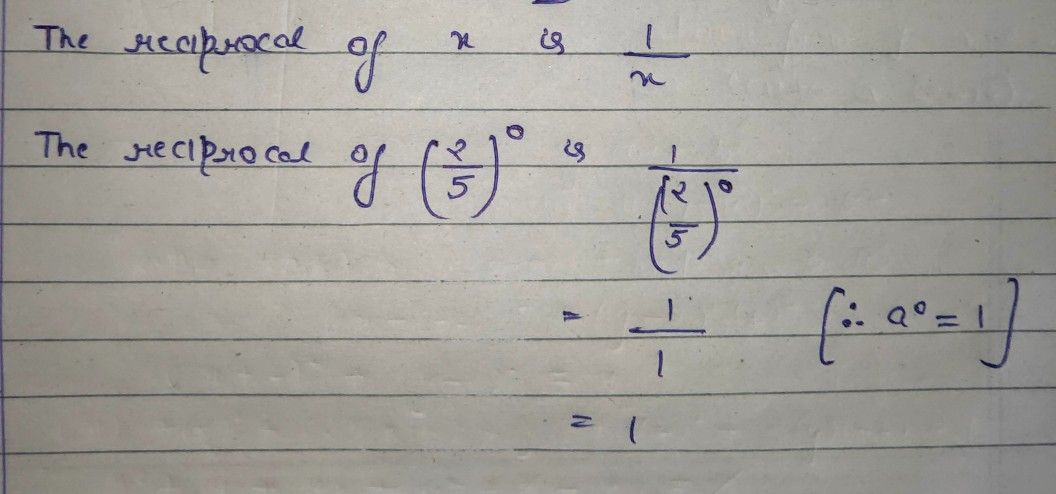Symbol
Problem$8$ $\left(1$ Point) $1\right)$ The\ reciprocal\\ $0+11\right)$ \left(\frac{2} $c\left(2\right)$ {5}\right)^0\ $\right)$ \ $1111s\right)$ $S$ $S1S$ $s3S$ $S4S$ $s2S$